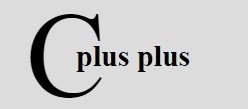# C++ Pointers and Objects

« Previous Tutorial Next Tutorial »

C++ allows you to have pointers to objects. The pointers pointing to objects are referred to as Object Pointers.

## C++ Declaration and Use of Object Pointers

Just like other pointers, the object pointers are declared by placing in front of a object pointer's name. It takes the following general form :

`class-name ∗ object-pointer ;`

where class-name is the name of an already defined class and object-pointer is the pointer to an object of this class type. For example, to declare optr as an object pointer of Sample class type, we shall write

`Sample ∗optr ;`

where Sample is already defined class. When accessing members of a class using an object pointer, the arrow operator (->) is used instead of dot operator.

The following program illustrates how to access an object given a pointer to it. This C++ program illustrates the use of object pointer

```/* C++ Pointers and Objects. Declaration and Use
* of Pointers. This program demonstrates the
* use of pointers in C++ */

#include<iostream.h>
#include<conio.h>
class Time
{
short int hh, mm, ss;
public:
Time()
{
hh = mm = ss = 0;
}
void getdata(int i, int j, int k)
{
hh = i;
mm = j;
ss = k;
}
void prndata(void)
{
cout<<"\nTime is "<<hh<<":"<<mm<<":"<<ss<<"\n";
}
};
void main()
{
clrscr();
Time T1, *tptr;
cout<<"Initializing data members using the object, with values 12, 22, 11\n";
T1.getdata(12,22,11);
cout<<"Printing members using the object ";
T1.prndata();
tptr = &T1;
cout<<"Printing members using the object pointer ";
tptr->prndata();
cout<<"\nInitializing data members using the object pointer, with values 15, 10, 16\n";
tptr->getdata(15, 10, 16);
cout<<"printing members using the object ";
T1.prndata();
cout<<"Printing members using the object pointer ";
tptr->prndata();
getch();
}```

On compiling and executing the above program, it will produce the following output :

The above program is self-explanatory. To access public members using an object the dot operator is used and to access public members using an object pointer the arrow operator is used.

As you know, when a pointer is incremented, it points to the next element of its type. The same is true of pointer to object.

## C++ this Pointer

As soon as you define a class, the member functions are created and placed in the memory space only once. That is, only one copy of member functions is maintained that is shared by all the objects of the class. Only space for data members is allocated separately for each object (See the following figure).

This has an associated problem. If only one instance of a member function exists, how does it come to know which object's data member is to be manipulated ? For example, if member function 3 is capable of changing the value of data member2 and we want to change the value of data member2 of object1. How could the member function3 come to know which object's data member2 is to be changed ?

The answer to this problem is this pointer. When a member function is called, it is automatically passed an implicit (in-built) argument that is a pointer to the object that invoked the function. This pointer is called this. That is if object1 is invoking member function3, then an implicit argument is passed to member function3 that points to object1 i.e., this pointer now points to object1. The this pointer can be thought of analogous to the ATM card. For instance, in a bank there are many accounts. The account holders can withdraw amount or view their bank-statements through Automatic-Teller-Machines. Now, these ATMs can withdraw from any account in the bank, but which account are they supposed to work upon ? This is resolved by the ATM card, which gives the identification of user and his accounts, from where the amount is withdrawn.

Similarly, the this pointer is the ATM cards for objects, which identifies the currently-calling object. The this pointer stores the address of currently-calling object. To understand this, consider the following example program (following program illustrates the functioning of this pointer) :

```/* C++ Pointers and Objects. The this pointer.
* This C++ program demonstrates about the this
* pointer in C++ */

#include<iostream.h>
#include<conio.h>
#include<string.h>
class Salesman
{
char name;
float total_sales;
public:
Salesman(char *s, float f)
{
strcpy(name, "");
strcpy(name, s);
total_sales = f;
}
void prnobject(void)
{
cout.write(this->name, 26);         // use of this pointer
cout<<" has invoked prnobject().\n";
}
};
void main()
{
clrscr();
Salesman Rajat("Rajat", 21450), Ravi("Ravi", 23190), Vikrant("Vikrant", 19142);
/* above statement creates three objects */
Rajat.prnobject();
Vikrant.prnobject();
Ravi.prnobject();
getch();
}```

Above C++ program will produce the following output :

It is obvious from the above output that when the object Rajat invokes the member function prnobject(), this points to Rajat and thus prints the name data member of Rajat. Similarly, this points to Vikrant and Ravi objects when they invoke prnobject().

Within a member function, the members of a class can be accessed directly, without any object or class qualification. Thus inside prnobject(), the statement :

`cout.write(this->name, 26) ;`

is same as

`cout.write(name,26);`

Remembers, the this pointer points to the object invoked prnobject(). Thus, this->name refers to that object's copy of name. The (this) refers to the object itself. With (*this), dot(.) operator should be used in order to access the member elements of the object as this is not a pointer to object, it is the object itself.

C++ Online Test

« Previous Tutorial Next Tutorial »